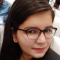## Rearrange positive and negative numbers in O(n) time and O(1) extra space in C++Sunidhi Bansal

Updated on 02-Nov-2021 06:48:28

198 Views

We are given an integer type array containing both positive and negative numbers, let's say, arr[] of any given size. The task is to rearrange an array in such a manner that all positive and negative numbers should be at alternate positions and if there are extra positive or negative ... Read More

## Rearrange array such that even positioned are greater than odd in C++Sunidhi Bansal

Updated on 02-Nov-2021 06:43:15

84 Views

We are given an integer type array containing both positive and negative numbers, let's say, arr[] of any given size. The task is to rearrange an array in such a manner that all the elements at an even position or index should be greater than the elements at an odd ... Read More

## Rearrange array such that even index elements are smaller and odd index elements are greater in C++Sunidhi Bansal

Updated on 02-Nov-2021 06:40:49

253 Views

We are given an integer type array containing both positive and negative numbers, let's say, arr[] of any given size. The task is to rearrange an array in such a manner that all the elements at an even position or index should be less than the elements at an odd ... Read More

## Rearrange array in alternating positive & negative items with O(1) extra space in C++Sunidhi Bansal

Updated on 02-Nov-2021 06:38:07

427 Views

We are given an integer type array containing both positive and negative numbers, let's say, arr[] of any given size. The task is to rearrange an array in such a manner that there will be a positive number that will be surrounded by negative numbers. If there are more positive ... Read More

## Rearrange an array to minimize sum of product of consecutive pair elements in C++Sunidhi Bansal

Updated on 02-Nov-2021 06:34:37

158 Views

We are given a positive integer type array, let's say, arr[] of any given size. The task is to rearrange an array in such a manner that when we multiply an element with its alternative element and then add all the resultant elements then it should return the minimum sum.Let ... Read More

## Rearrange an array such that every odd indexed element is greater than its previous in C++Sunidhi Bansal

Updated on 02-Nov-2021 06:31:03

93 Views

We are given a positive integer type array, let's say, arr[] of any given size. The task is to rearrange an array in such a manner that all the elements present at an odd index should value greater than the element presents at an even index and print the result.Let ... Read More

## Rearrange an array such that arr[i] = i in C++Sunidhi Bansal

Updated on 02-Nov-2021 06:27:57

209 Views

We are given a positive integer type array, let's say, arr[] of any given size such that elements in an array should value greater than 0 but less than the size of an array. The task is to rearrange an array in such a manner that if arr[i] is ‘i’, ... Read More

## Rearrange an array such that ‘arr[j]’ becomes ‘i’ if ‘arr[i]’ is ‘j’ in C++Sunidhi Bansal

Updated on 02-Nov-2021 06:20:09

157 Views

We are given a positive integer type array, let's say, arr[] of any given size such that elements in an array should value greater than 0 but less than the size of an array. The task is to rearrange an array in such a manner that if arr[j] is ‘j’ ... Read More

## Rearrange an array so that arr[i] becomes arr[arr[i]] with O(1) extra space using C++Sunidhi Bansal

Updated on 02-Nov-2021 06:16:33

506 Views

We are given a positive integer type array, let's say, arr[] of any given size such that elements in an array should value greater than 0 but less than the size of an array. The task is to rearrange an array in such a manner that arr[i] becomes arr[arr[i]] within ... Read More

## C++ program to rearrange an array in maximum minimum formSunidhi Bansal

Updated on 02-Nov-2021 06:11:54

1K+ Views

We are given an integer array which can be arranged in sorted/unsorted manner. The task is to first sort the array if the values are unsorted then arrange the array in such a manner that the first element of array will be the maximum value, second will be the minimum ... Read More

Previous 1 ... 5 6 7 8 9 ... 111 Next